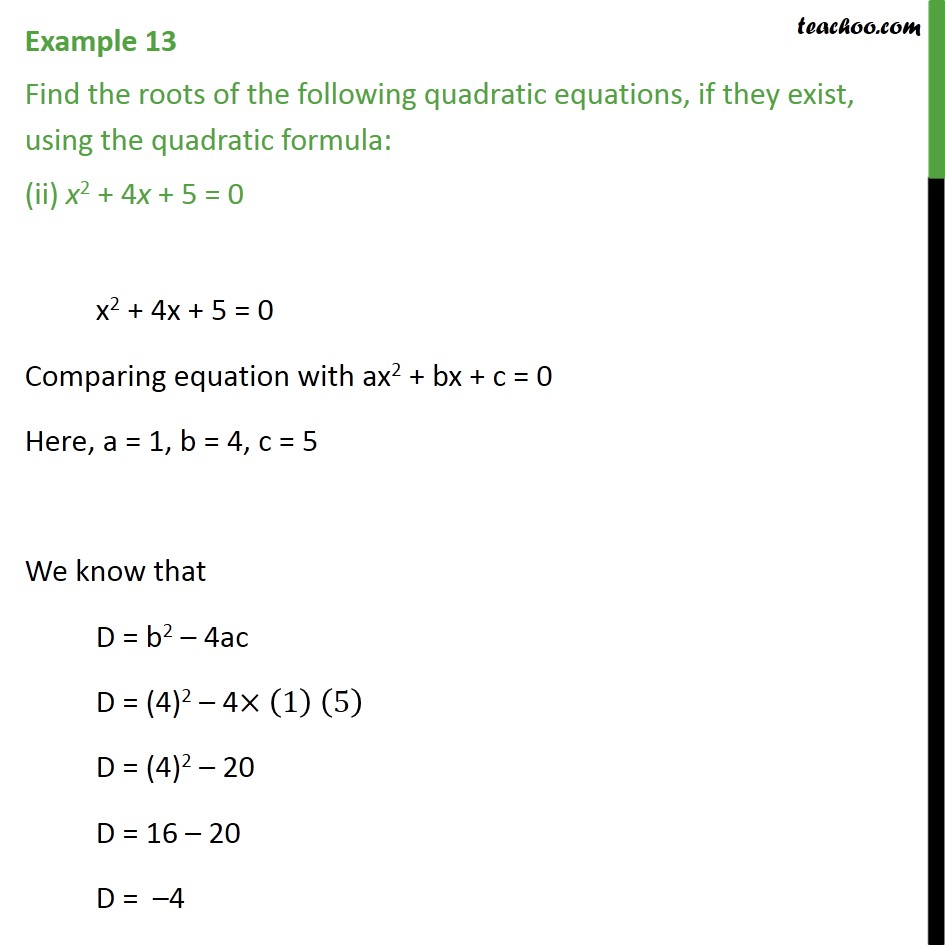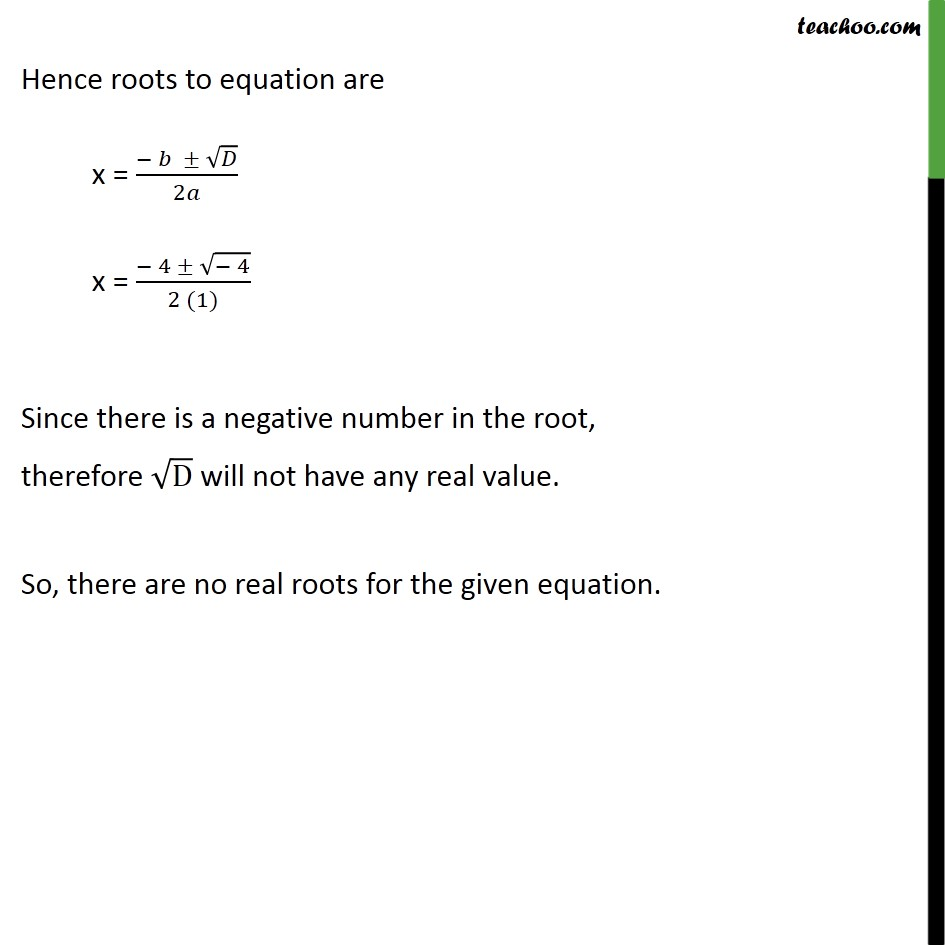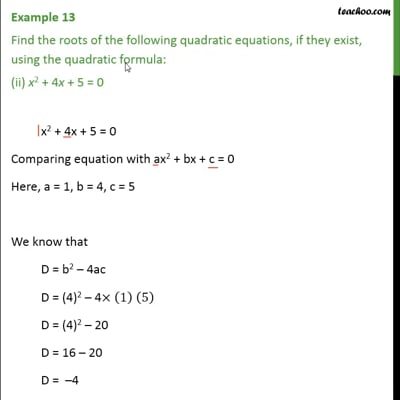Examples

Chapter 4 Class 10 Quadratic Equations
Serial order wiseThis video is only available for Teachoo black users

Maths Crash Course - Live lectures + all videos + Real time Doubt solving!

### Transcript

Example 13 Find the roots of the following quadratic equations, if they exist, using the quadratic formula: (ii) x2 + 4x + 5 = 0 x2 + 4x + 5 = 0 Comparing equation with ax2 + bx + c = 0 Here, a = 1, b = 4, c = 5 We know that D = b2 4ac D = (4)2 4 (1) (5) D = (4)2 20 D = 16 20 D = 4 Hence roots to equation are x = ( )/2 x = ( 4 ( 4))/(2 (1)) Since there is a negative number in the root, therefore D will not have any real value. So, there are no real roots for the given equation.